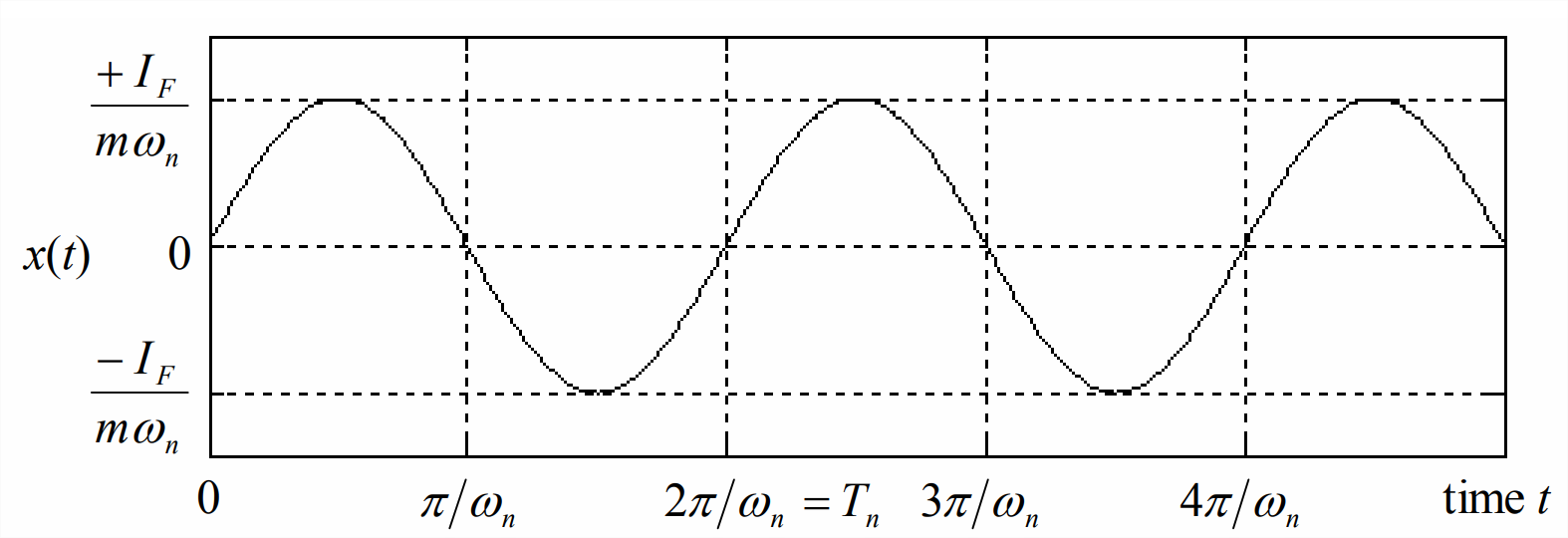# 8.7: Ideal Impulse Response of an Undamped Second Order System

The ODE for a standard undamped 2nd order system is $$\ddot{x}+\omega_{n}^{2} x=\omega_{n}^{2} u(t)$$. Let the input function be the ideal impulse, $$u(t)=I_{U} \delta(t)$$, and let the pre-impulse initial conditions be $$x\left(0^{-}\right) \equiv x_{0}$$, $$\dot{x}\left(0^{-}\right) \equiv \dot{x}_{0}$$. Just as for the 1st order system with ideal-impulse excitation in Section 8.5, we will solve the present problem by the conventional Laplace-transform approach. Note that we will use the second derivative form of Equation 8.6.4: $$L[\ddot{f}]=s^{2} F(s)-s f\left(0^{-}\right)-\dot{f}\left(0^{-}\right)$$. With $$L[\delta(t)]=1$$ from Equation 8.4.6, the steps of the solution are:

$L[\mathrm{ODE}]: \quad s^{2} X(s)-s x_{0}-\dot{x}_{0}+\omega_{n}^{2} X(s)=b U(s)=\omega_{n}^{2} I_{U} \times 1$

$\Rightarrow \quad X(s)=\frac{\omega_{n}^{2} I_{U}+\dot{x}_{0}+s x_{0}}{s^{2}+\omega_{n}^{2}}=\left(\omega_{n} I_{U}+\frac{\dot{x}_{0}}{\omega_{n}}\right) \frac{\omega_{n}}{s^{2}+\omega_{n}^{2}}+x_{0} \frac{s}{s^{2}+\omega_{n}^{2}}\label{eqn:8.25}$

$\Rightarrow \quad x(t)=\left(\omega_{n} I_{U}+\frac{\dot{x}_{0}}{\omega_{n}}\right) \sin \omega_{n} t+x_{0} \cos \omega_{n} t, t \geq 0^{+}\label{eqn:8.26}$

At the risk of being excessively repetitive, we emphasize that the discussions in Sections 8.5 and 8.6 show that response Equation $$\ref{eqn:8.26}$$ is the post-impulse solution, certainly valid mathematically for $$t \geq 0^{+}$$, but not necessarily correct, in particular, for the pre-impulse initial conditions at $$t=0^-$$.

Solution Equation $$\ref{eqn:8.26}$$ holds for a general undamped 2nd order system; let us adapt it specifically to a mass-spring system, with zero initial conditions, that is hit by an ideal impulse of force having magnitude $$I_{F}$$, with units, for example, of N-s or lb-s. From Equation 7.1.4, we find

$u(t)=f_{x}(t) / k \Rightarrow I_{U} \delta(t)=I_{F} \delta(t) / k \Rightarrow I_{U}=I_{F} / k\label{eqn:8.27}$

So, for translational motion $$x(t)$$ of the mass, Equation $$\ref{eqn:8.26}$$ with zero initial conditions becomes (recall $$k=m \omega_{n}^{2}$$)

$x(t)=\omega_{n} \frac{I_{F}}{k} \sin \omega_{n} t=\frac{I_{F}}{m \omega_{n}} \sin \omega_{n} t\label{eqn:8.28}$

Figure $$\PageIndex{1}$$ shows a few cycles of response Equation $$\ref{eqn:8.28}$$.Figure $$\PageIndex{1}$$: Ideal impulse response of a mass-spring system